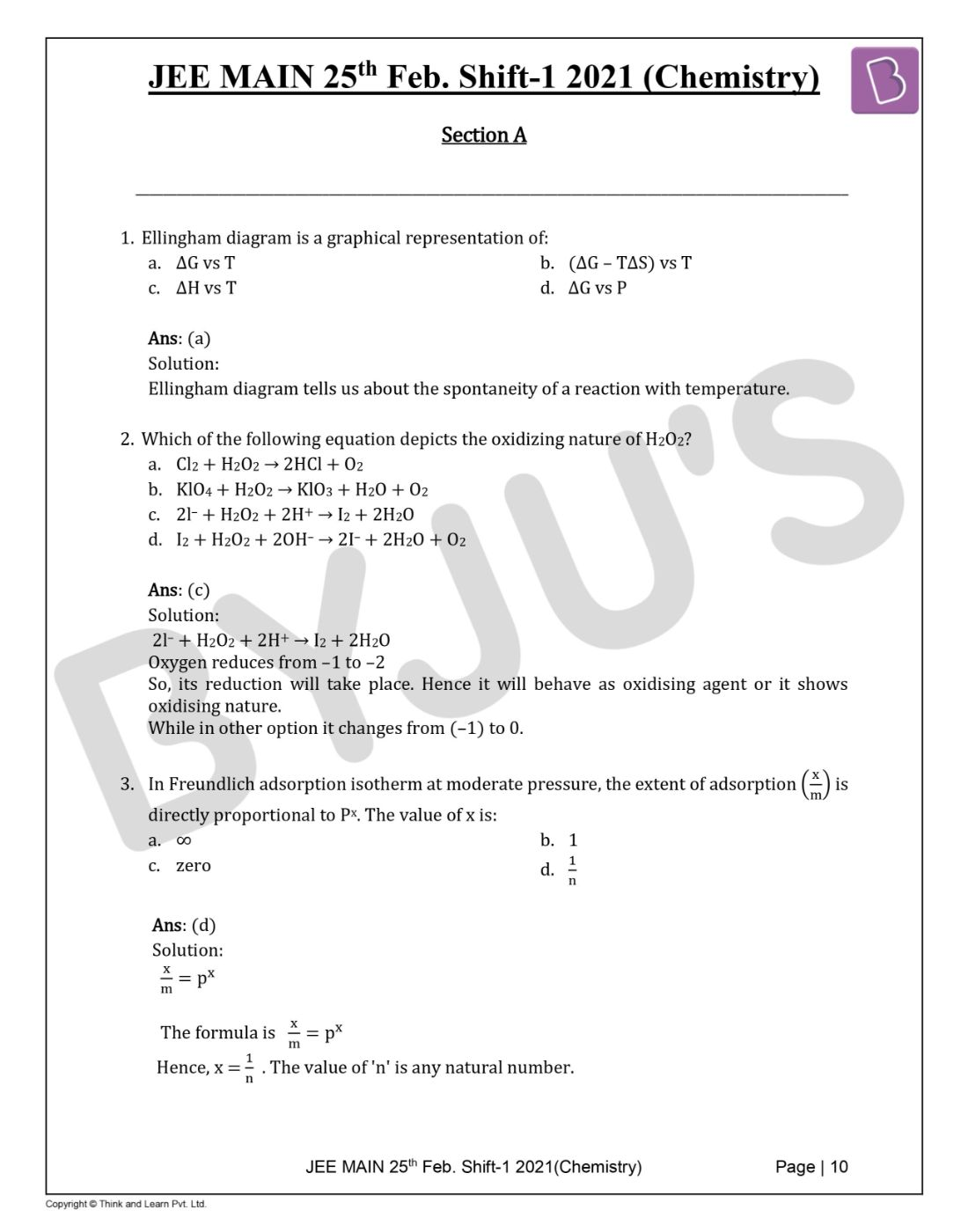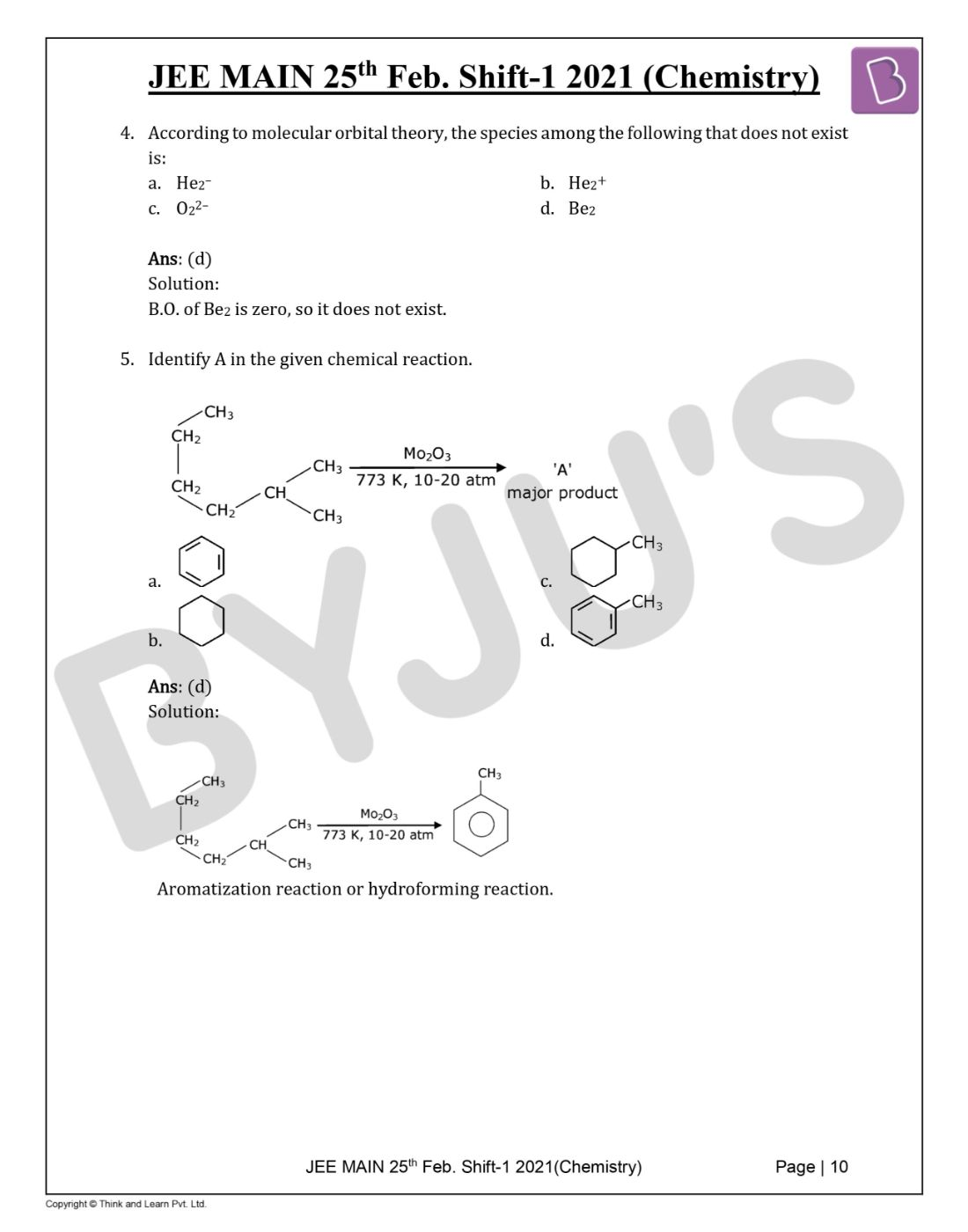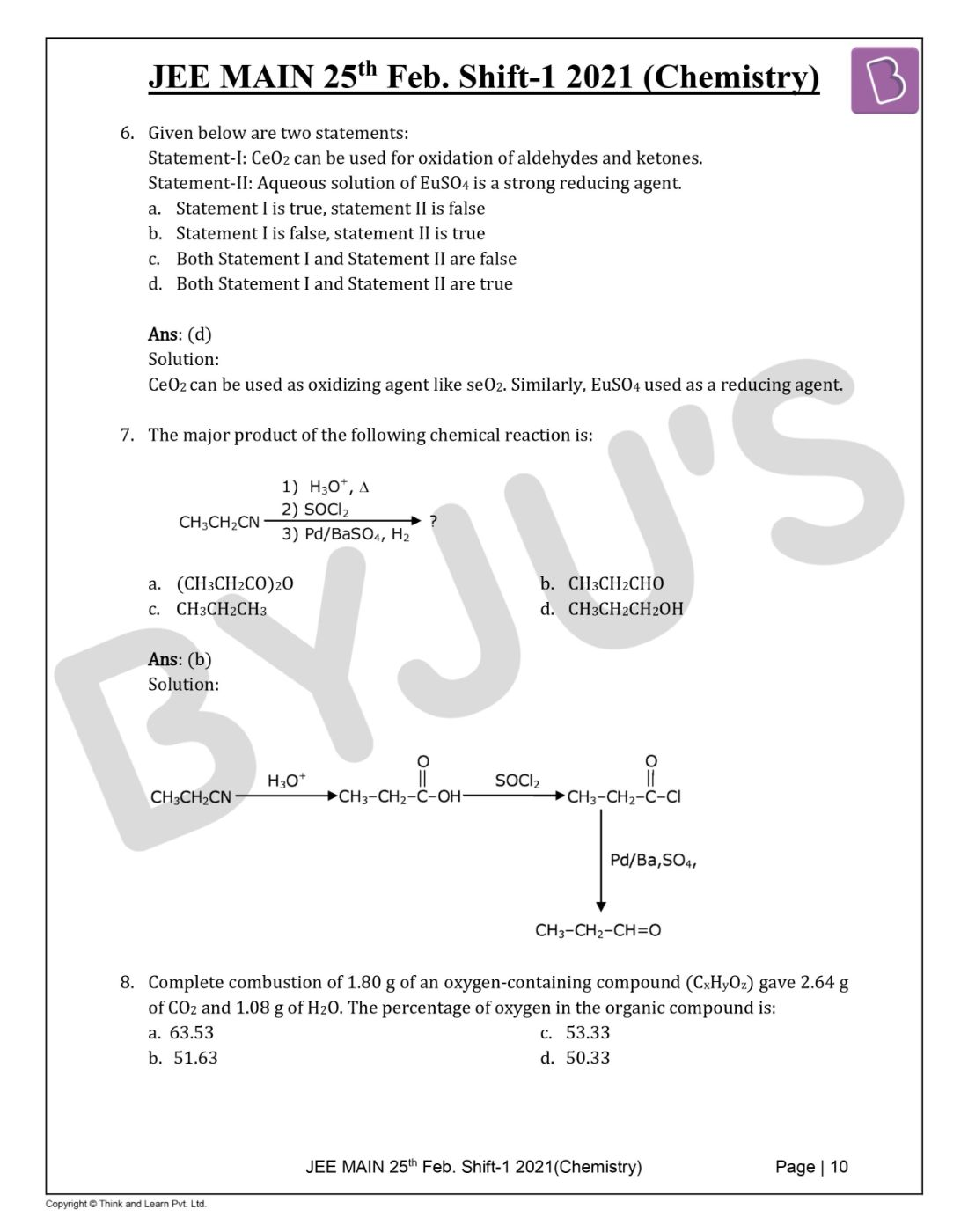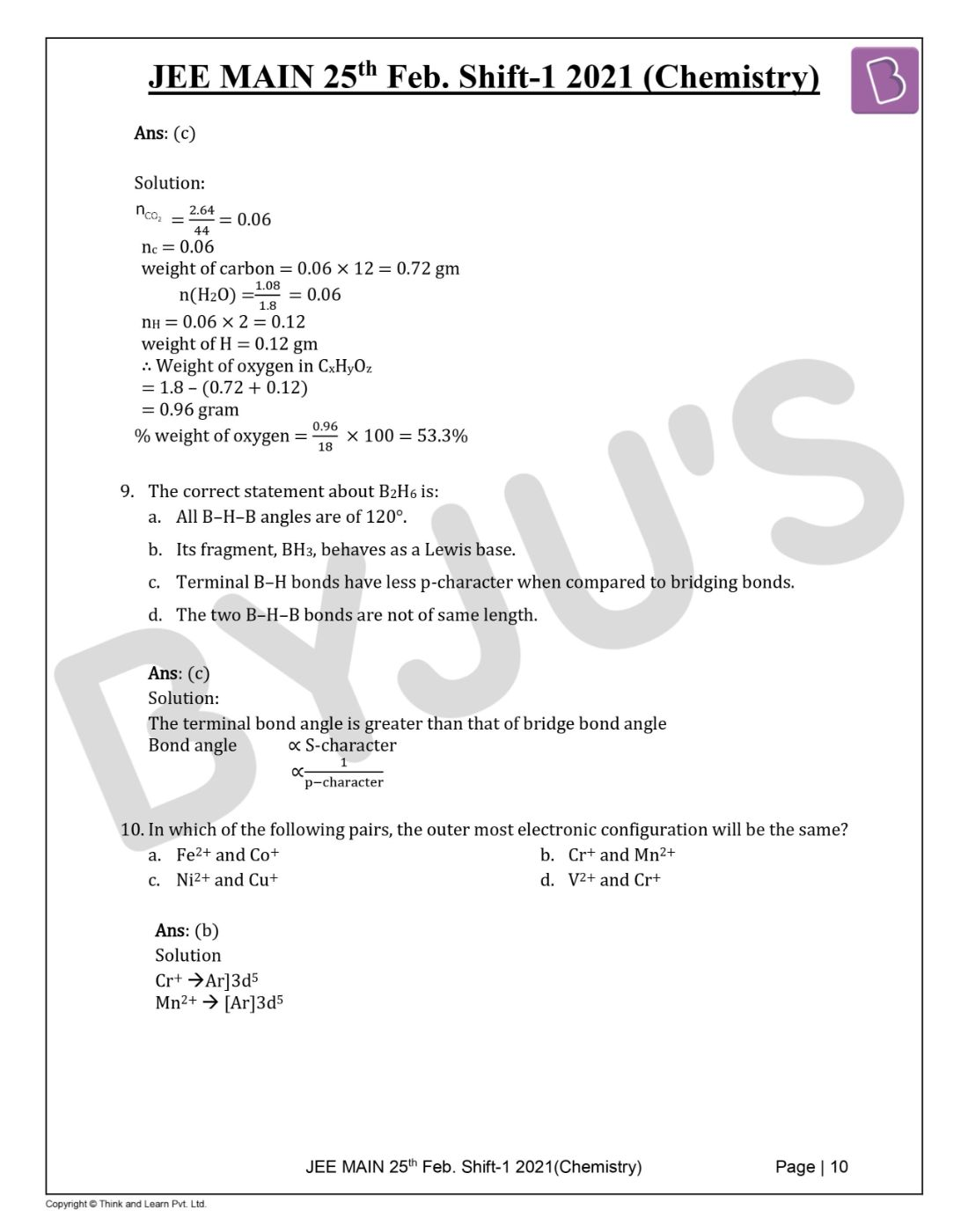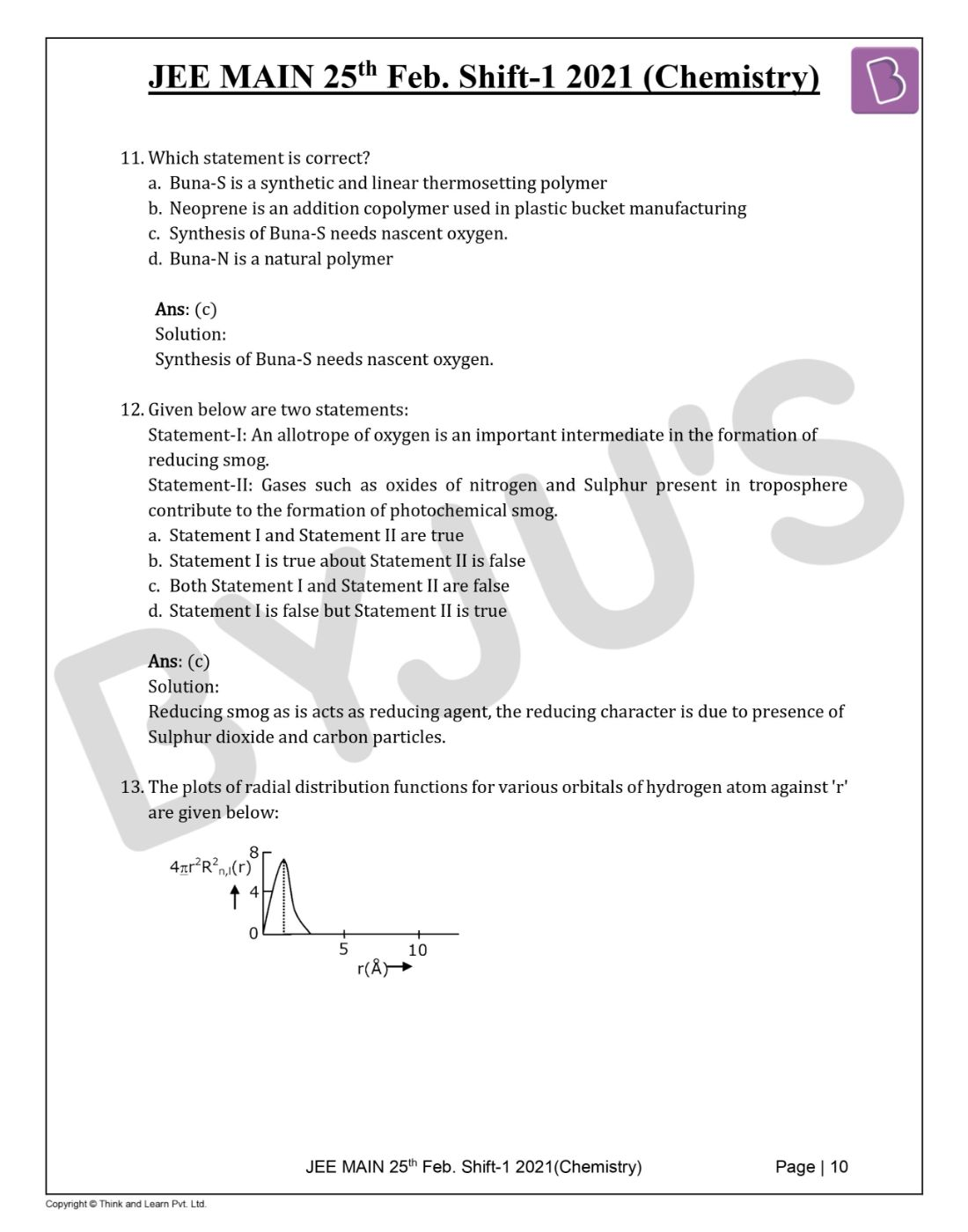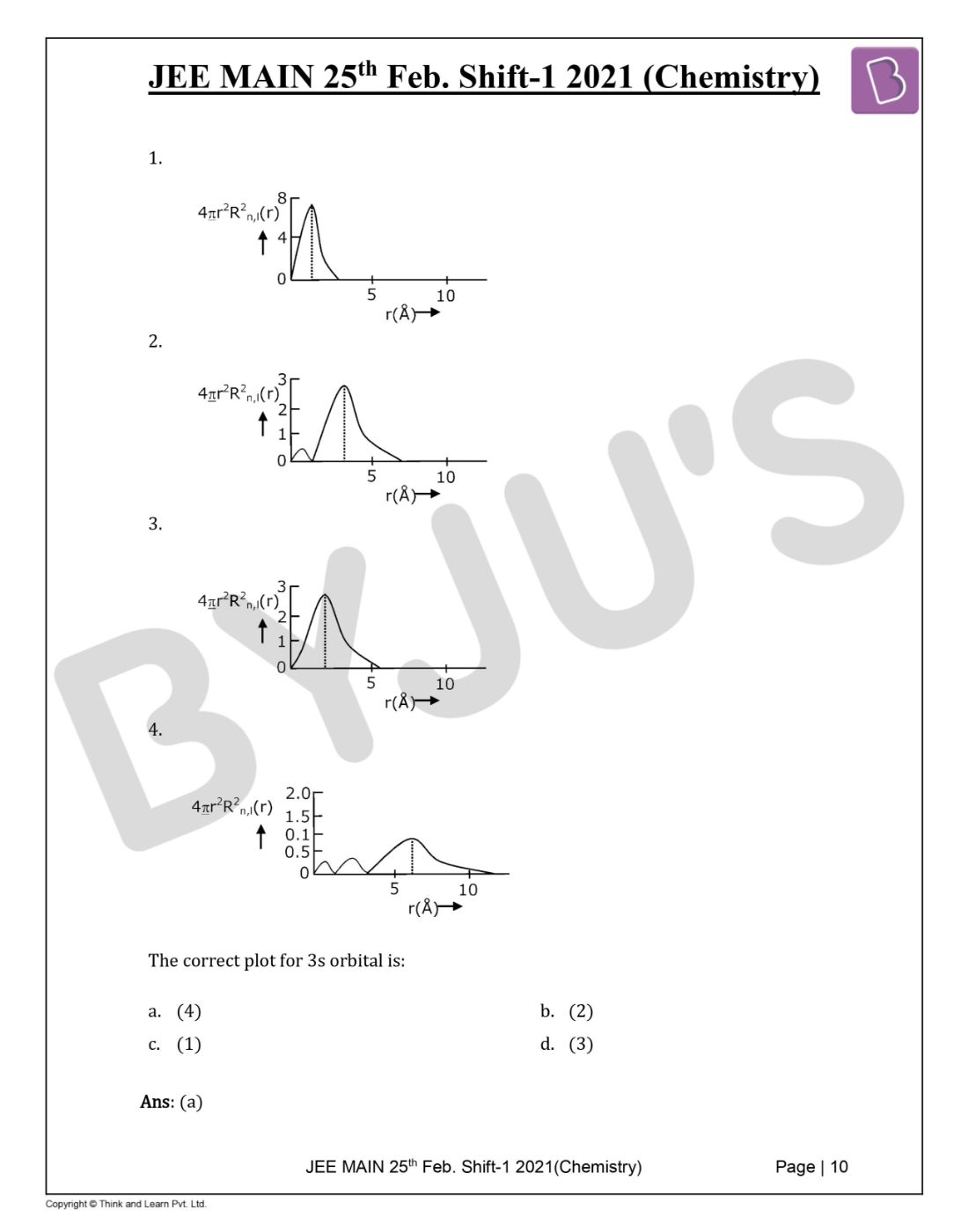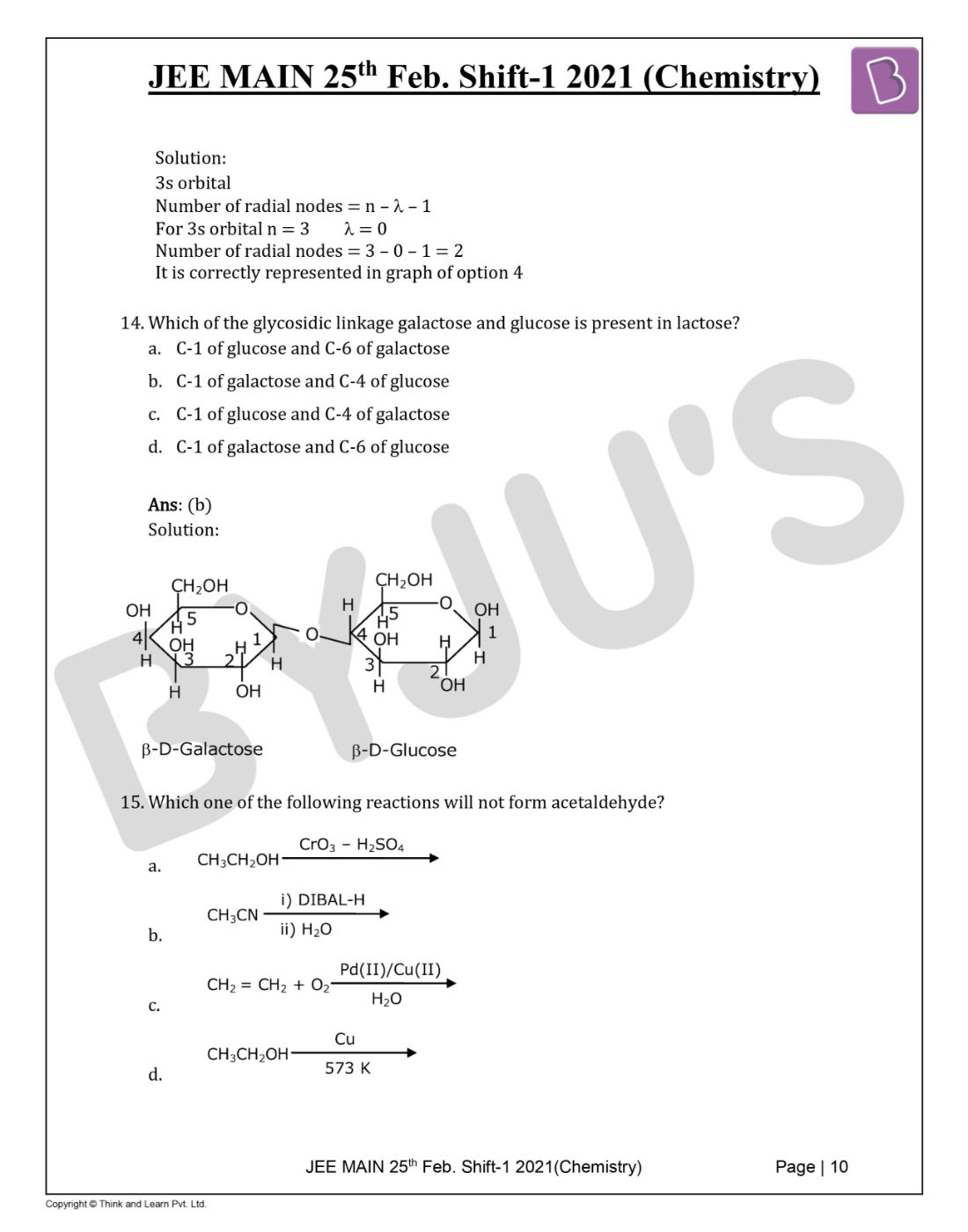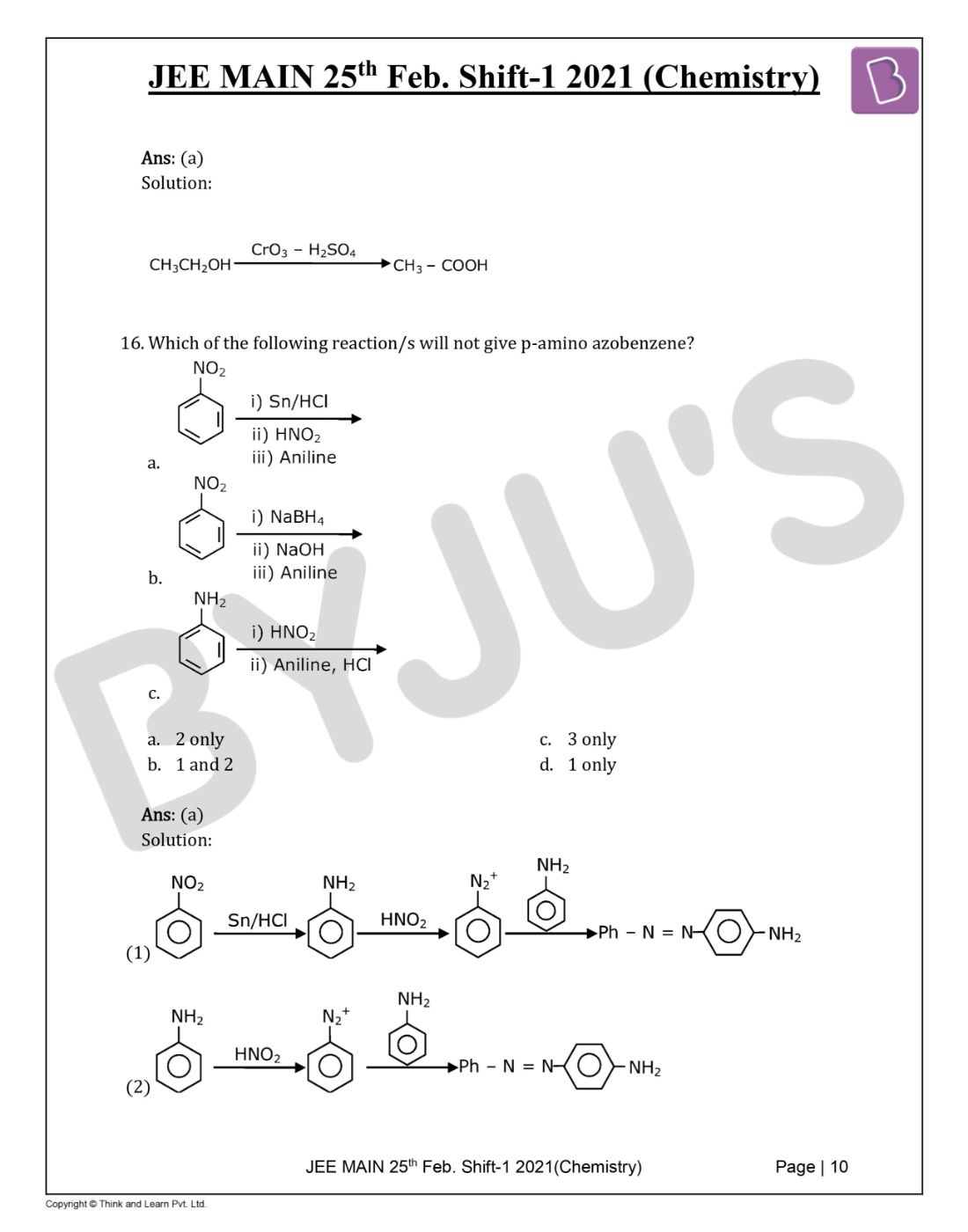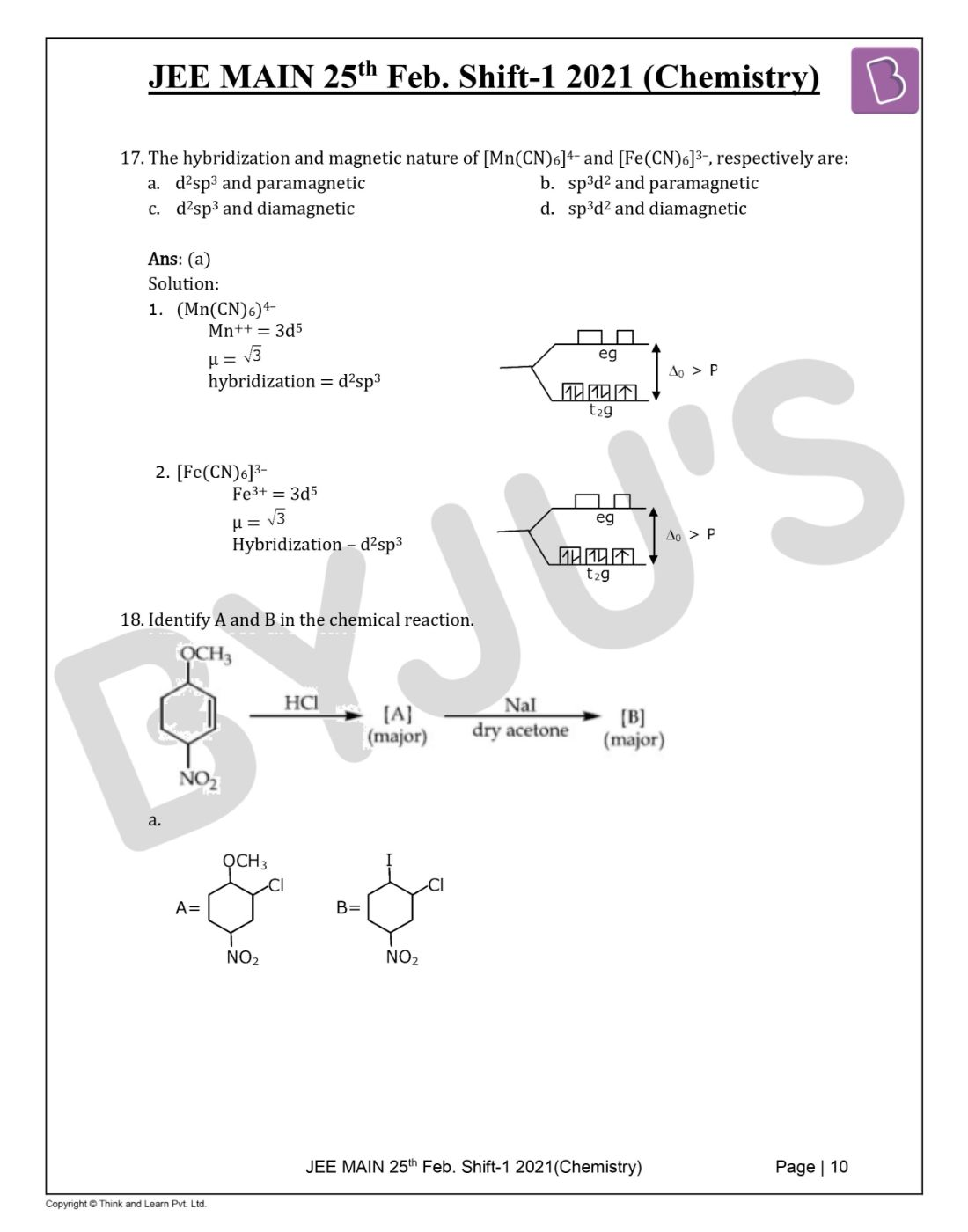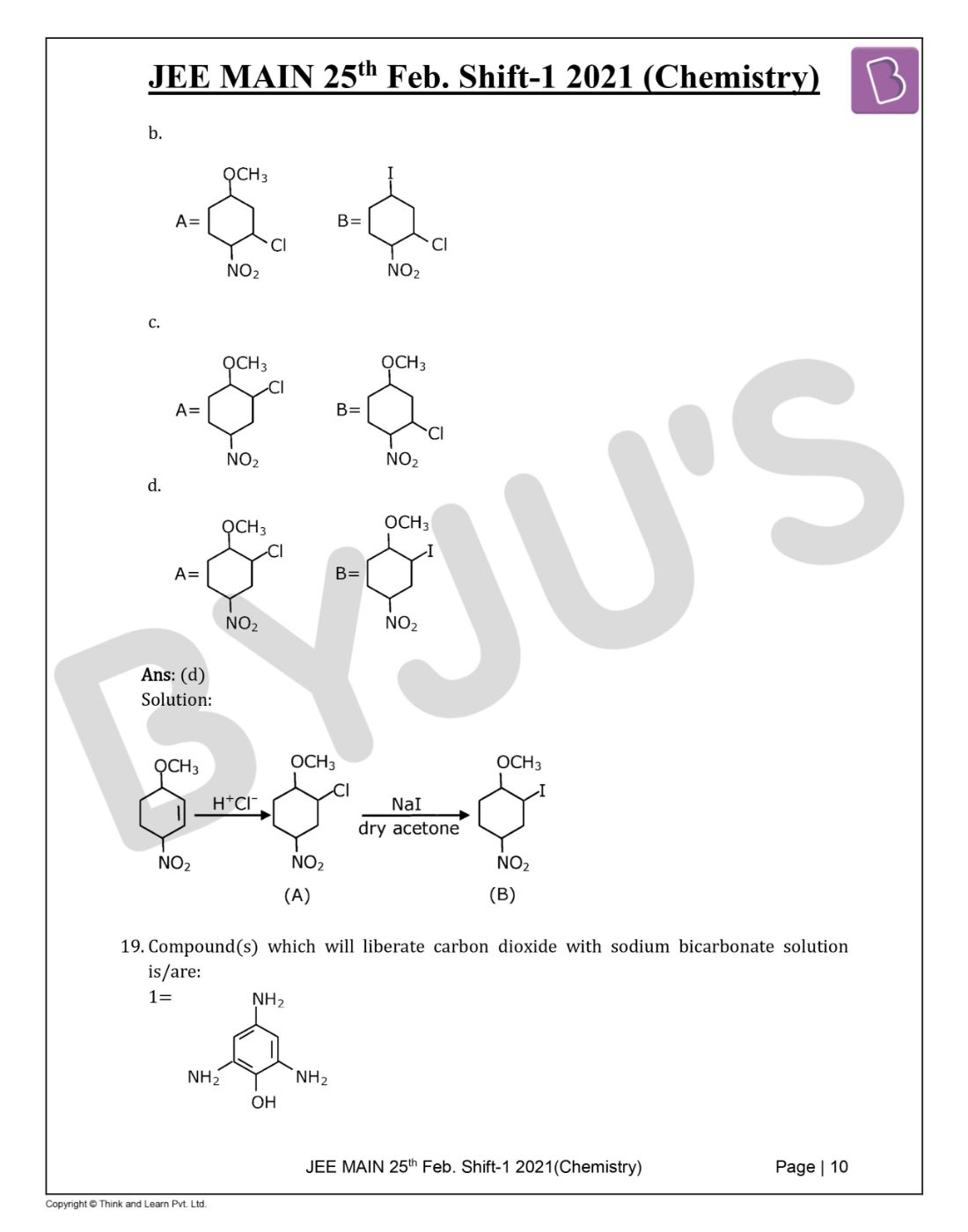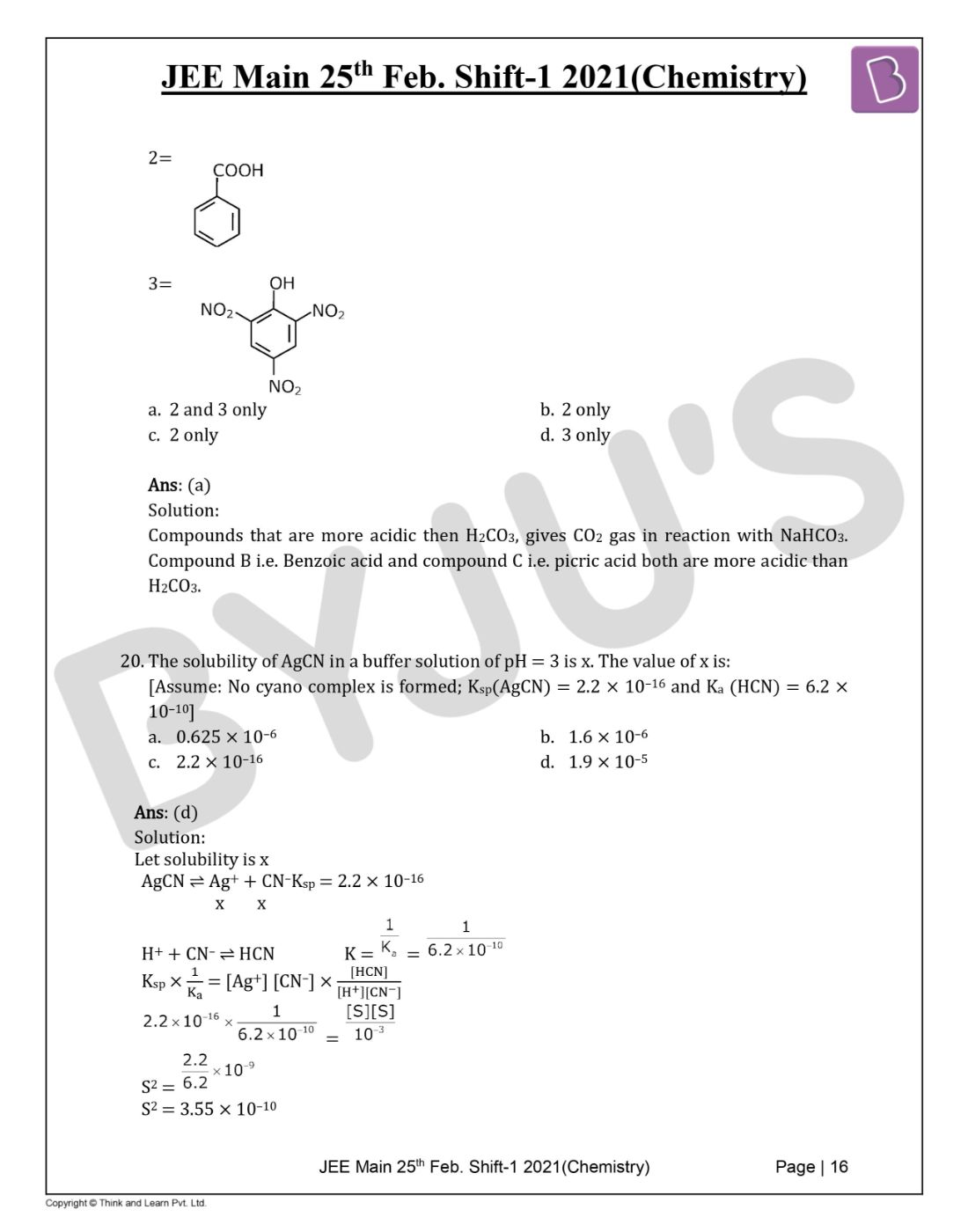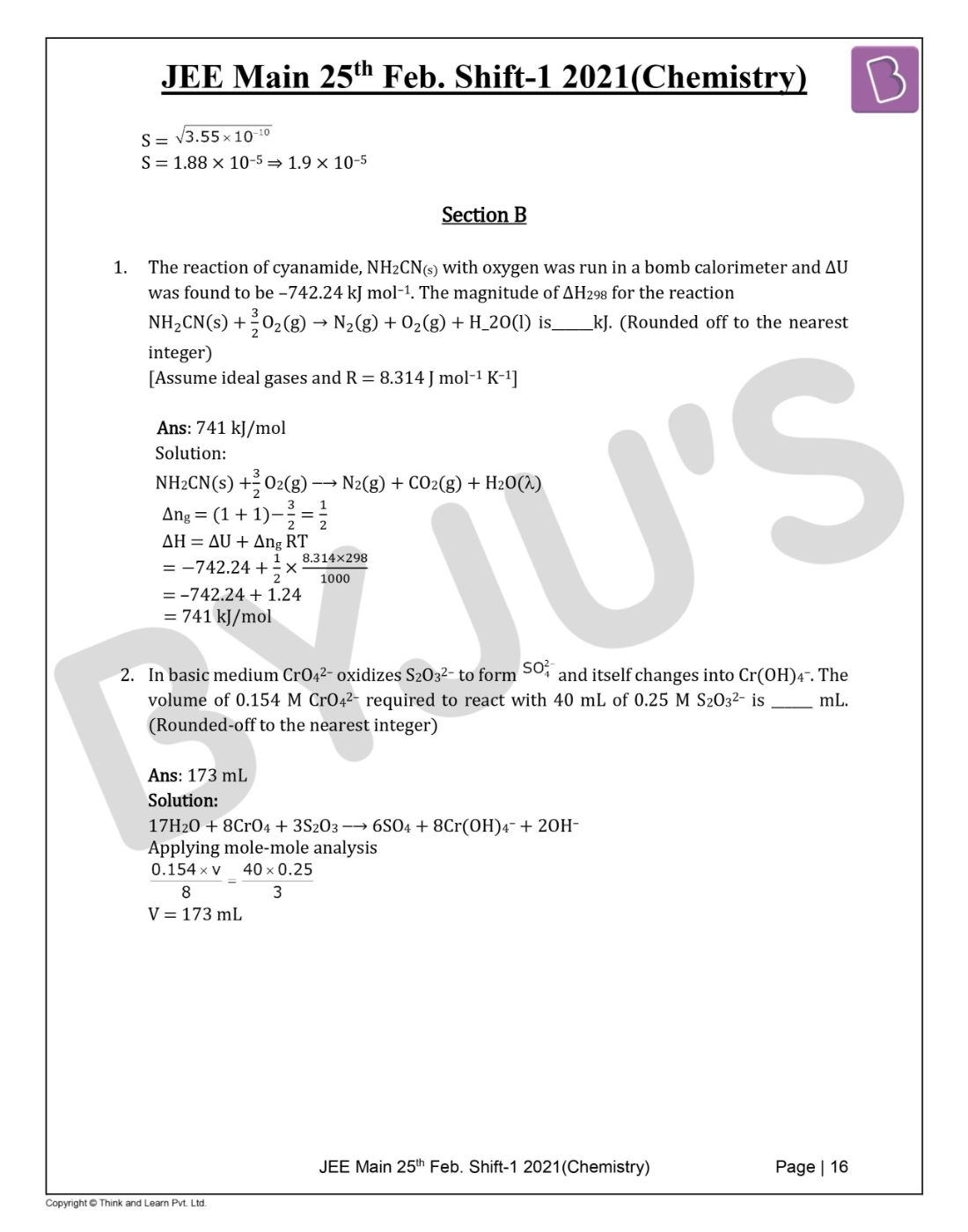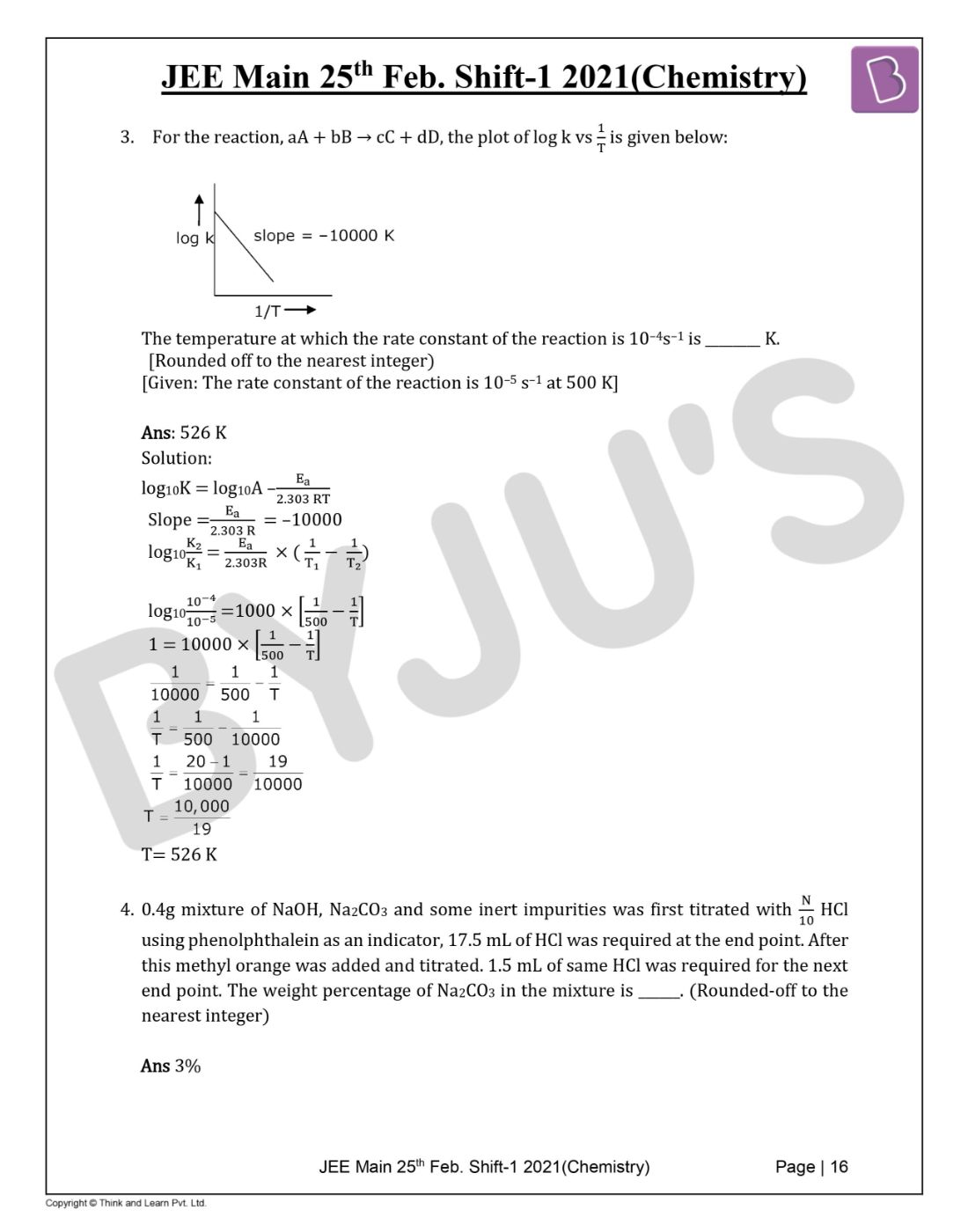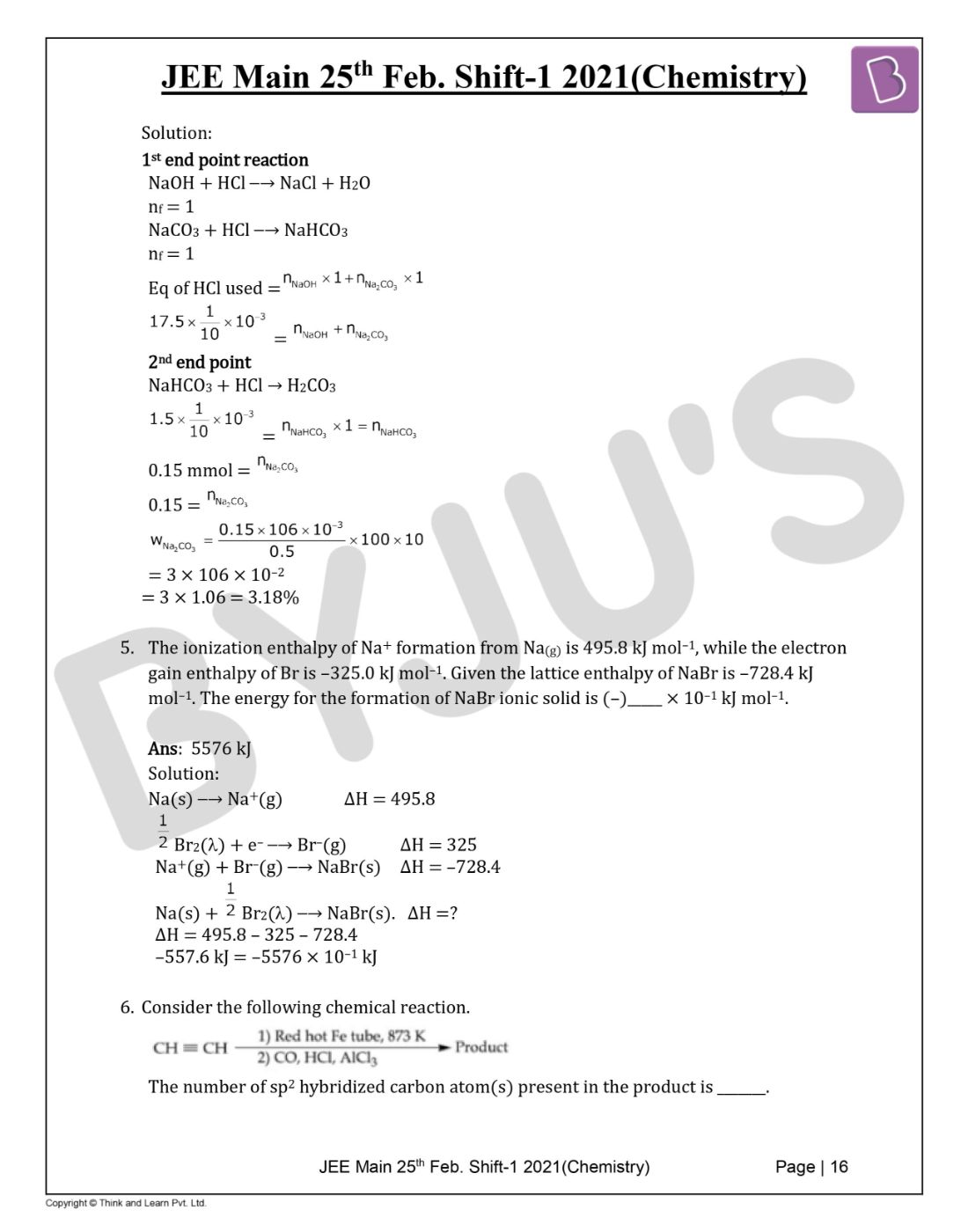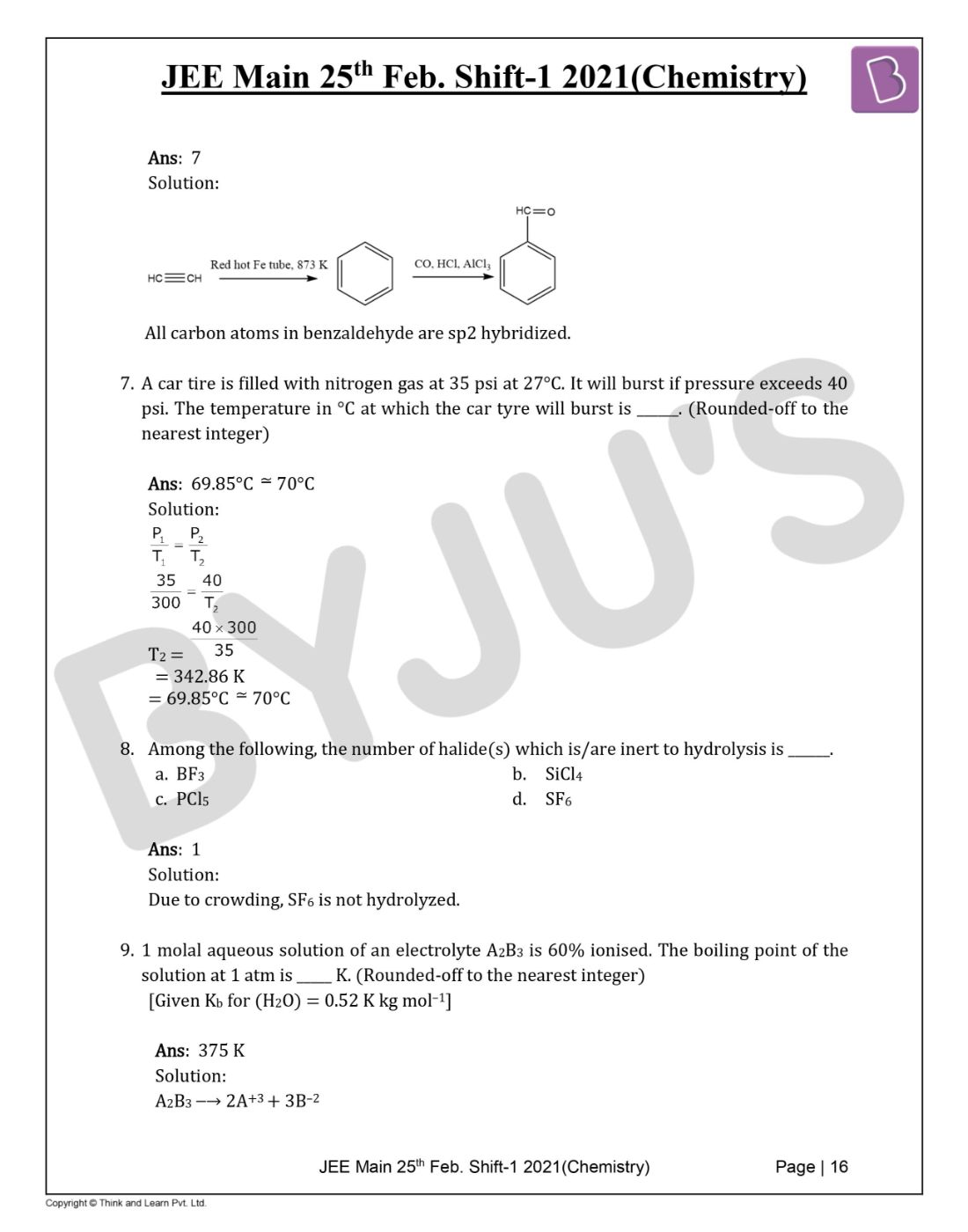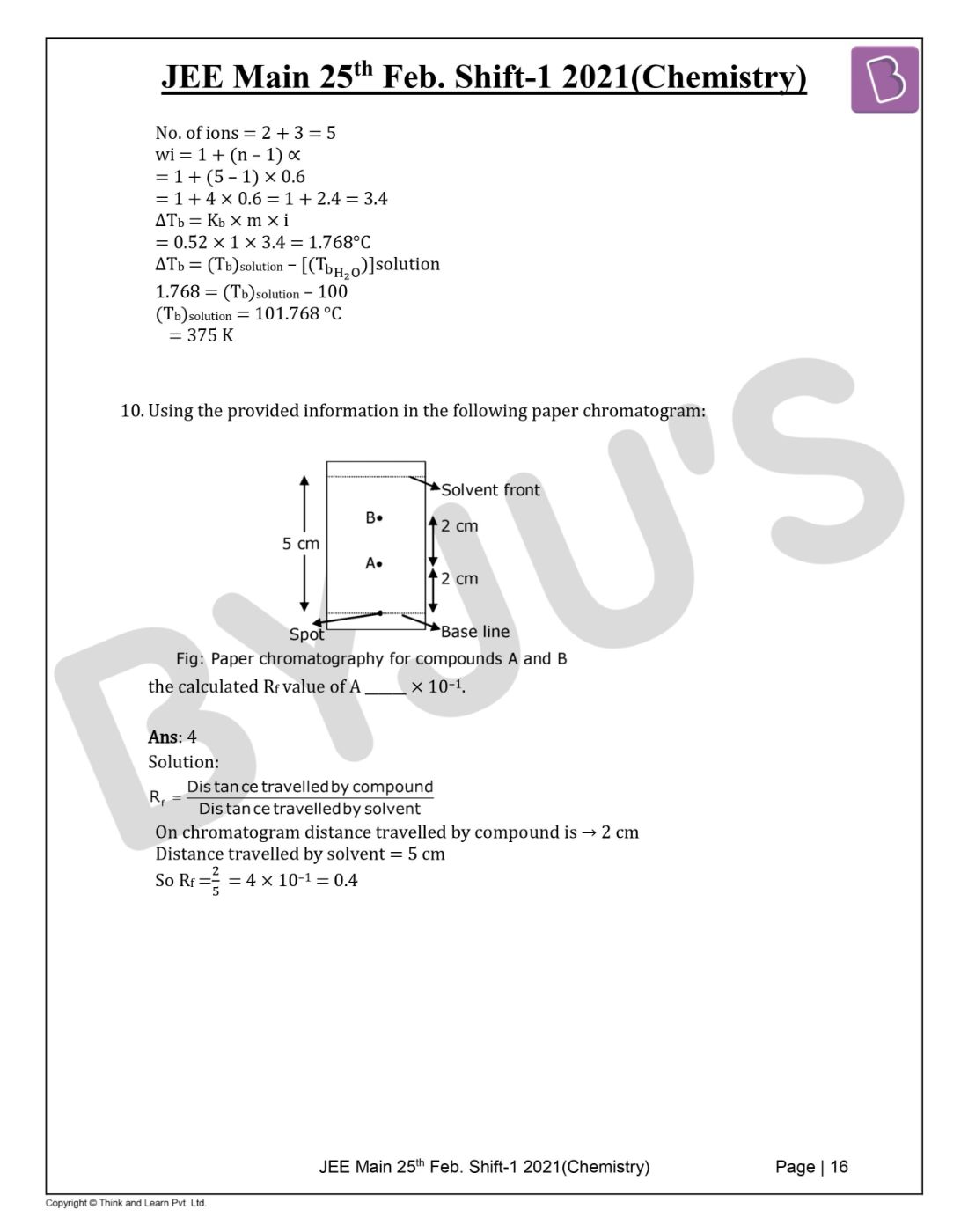Win up to 100% scholarship on Aakash BYJU'S JEE/NEET courses with ABNAT Win up to 100% scholarship on Aakash BYJU'S JEE/NEET courses with ABNAT

# JEE Main 2021 Chemistry Paper With Solutions Feb 25 Shift 1

Students will find the solved JEE Main 2021 Chemistry question paper for Feb 25 Shift 1 session. Students can go through the question paper and find out what type of questions were asked in JEE main as well as the overall exam pattern, difficulty level and more. Additionally, this paper can prove to be a good resource for preparing effectively for the upcoming examination. Nonetheless, students can view directly or download the question paper PDF instantly from this page.

The solutions for each question have been prepared by our subject matter experts and they have provided detailed explanations so that students will find it easier to learn and understand each topic covered in the question paper. All in all, the JEE Main 2021 Chemistry question paper for Feb 25 Shift 1 is a good resource for studying productively for the upcoming examination.

JEE Main 2021 25th February Shift 1 Chemistry Question Paper

SECTION A

Question 1. Ellingham diagram is a graphical representation of:

a. ΔG vs T
b. (ΔG – TΔS) vs T
c. ΔH vs T
d. ΔG vs P

Ellingham diagram tells us about the spontaneity of a reaction with temperature.

Question 2. Which of the following equations depicts the oxidizing nature of H2O2?

a. Cl2 + H2O2 → 2HCl + O2
b. KlO4 + H2O2 → KlO3 + H2O + O2
c. 2l + H2O2 + 2H+ → I2 + 2H2O
d. I2 + H2O2 + 2OH → 2I + 2H2O + O2

2l + H2O2 + 2H+ → I2 + 2H2O

Oxygen reduces from –1 to –2

So, its reduction will take place. Hence it will behave as oxidising agent or it shows

oxidising nature.

While in other options it changes from (–1) to 0.

Question 3. In Freundlich adsorption isotherm at moderate pressure, the extent of adsorption (x/m) is directly proportional to Px. The value of x is:

a. ∞
b. 1
c. zero
d. 1/n

x / m = px

The formula is x / m = px

Hence, x= 1 / n. The value of ‘n’ is any natural number.

Question 4. According to molecular orbital theory, the species among the following that does not exist is:

a. He2
b. He2+
c. O22-
d. Be2

B.O. of Be2 is zero, so it does not exist.

Question 5. Identify A in the given chemical reaction.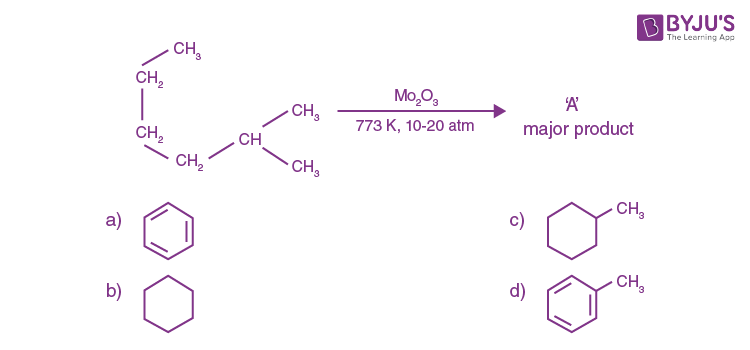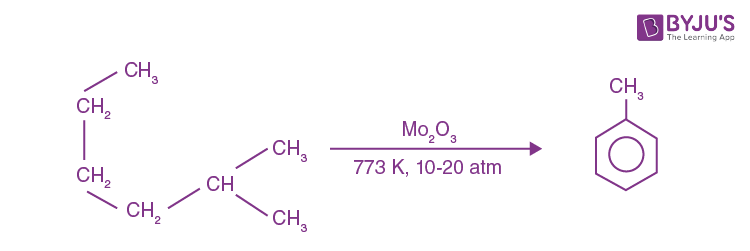Aromatization reaction or hydroforming reaction.

Question 6. Given below are two statements:

Statement-I: CeO2 can be used for oxidation of aldehydes and ketones.

Statement-II: Aqueous solution of EuSO4 is a strong reducing agent.

a. Statement I is true, statement II is false
b. Statement I is false, statement II is true
c. Both Statement I and Statement II are false
d. Both Statement I and Statement II are true

CeO2 can be used as an oxidizing agent like seO2. Similarly, EuSO4 is used as a reducing agent.

Question 7. The major product of the following chemical reaction is:a. (CH3CH2CO)2O
b. CH3CH2CHO
c. CH3CH2CH3
d. CH3CH2CH2OH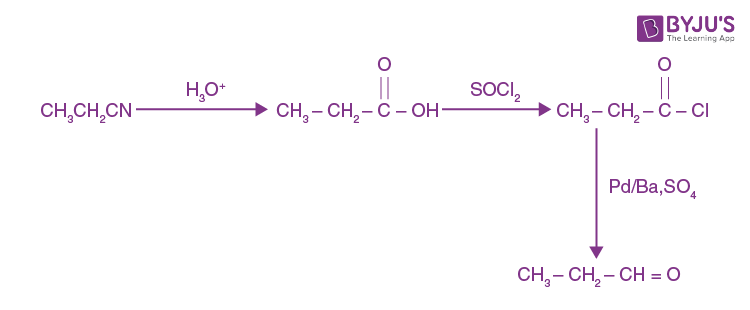Question 8. Complete combustion of 1.80 g of an oxygen-containing compound (CxHyOz) gave 2.64 g of CO2 and 1.08 g of H2O. The percentage of oxygen in the organic compound is:

a. 63.53
b. 51.63
c. 53.33
d. 50.33

nco2 = 2.64 / 44 = 0.06

nc = 0.06

weight of carbon = 0.06 × 12 = 0.72 gm

n(H2O) = 1.08 / 1.8 = 0.06

nH = 0.06 × 2 = 0.12

weight of H = 0.12 gm

∴ Weight of oxygen in CxHyOz

= 1.8 – (0.72 + 0.12)

= 0.96 gram

% weight of oxygen = 0.96/18 × 100 = 53.3%

Question 9.The correct statement about B2H6 is:

a. All B–H–B angles are of 120°.
b. Its fragment, BH3, behaves as a Lewis base.
c. Terminal B–H bonds have less p-character when compared to bridging bonds.
d. The two B–H–B bonds are not of the same length.

The terminal bond angle is greater than that of bridge bond angle

Bond angle ∝ S-character

$$\begin{array}{l}\alpha \frac{1}{p-character}\end{array}$$

Question 10. In which of the following pairs, the outermost electronic configuration will be the same?

a. Fe2+ and Co+
b. Cr+ and Mn2+
c. Ni2+ and Cu+
d. V2+ and Cr+

Cr+ → Ar]3d5

Mn2+ → [Ar]3d5

Question 11. Which statement is correct?

a. Buna-S is a synthetic and linear thermosetting polymer
b. Neoprene is an addition copolymer used in plastic bucket manufacturing
c. Synthesis of Buna-S needs nascent oxygen
d. Buna-N is a natural polymer

Synthesis of Buna-S needs nascent oxygen.

Question 12. Given below are two statements:

Statement-I: An allotrope of oxygen is an important intermediate in the formation of reducing smog.

Statement-II: Gases such as oxides of nitrogen and Sulphur present in the troposphere contribute to the formation of photochemical smog.

a. Statement I and Statement II are true
b. Statement I is true about Statement II is false
c. Both Statement I and Statement II are false
d. Statement I is false but Statement II is true

Reducing smog acts as a reducing agent and the reducing character is due to presence of sulphur dioxide and carbon particles.

Question 13. The plots of radial distribution functions for various orbitals of hydrogen atom against ‘r’ are given below: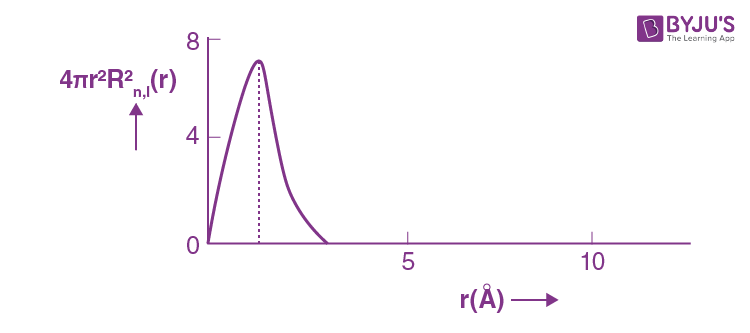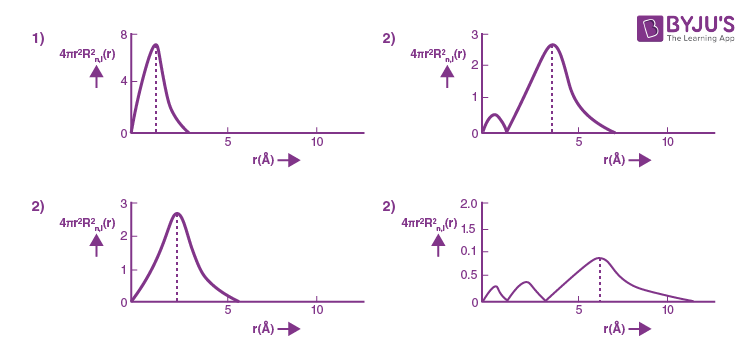The correct plot for 3s orbital is

a. (4)
b. (2)
c. (1)
d. (3)

3s orbital

Number of radial nodes = n – λ – 1

For 3s orbital n = 3, λ = 0

Number of radial nodes = 3 – 0 – 1 = 2

It is correctly represented in graph of option 4

Question 14. Which of the glycosidic linkage galactose and glucose is present in lactose?

a. C-1 of glucose and C-6 of galactose
b. C-1 of galactose and C-4 of glucose
c. C-1 of glucose and C-4 of galactose
d. C-1 of galactose and C-6 of glucose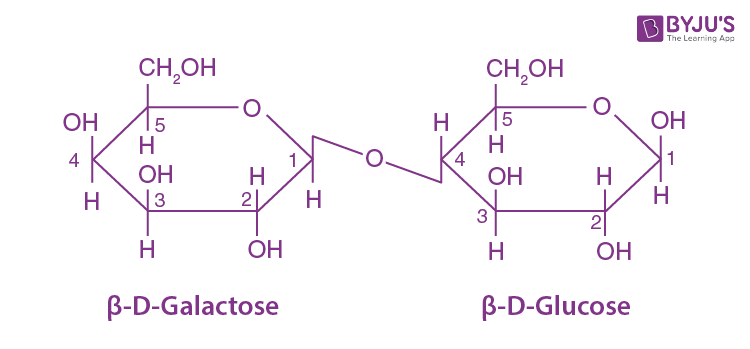Question 15. Which one of the following reactions will not form acetaldehyde?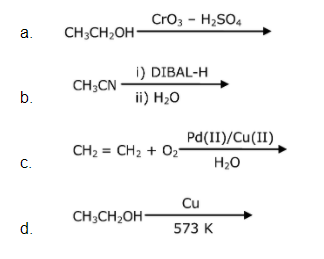Question 16. Which of the following reaction/s will not give p-amino azobenzene?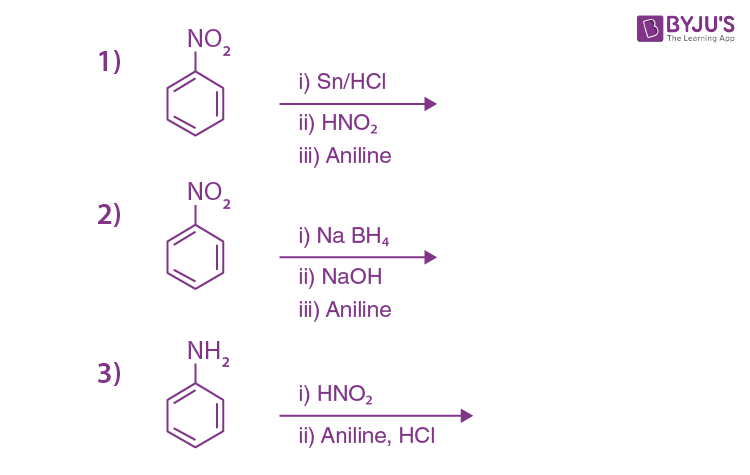a. 2 only
b. 1 and 2
c. 3 only
d. 1 only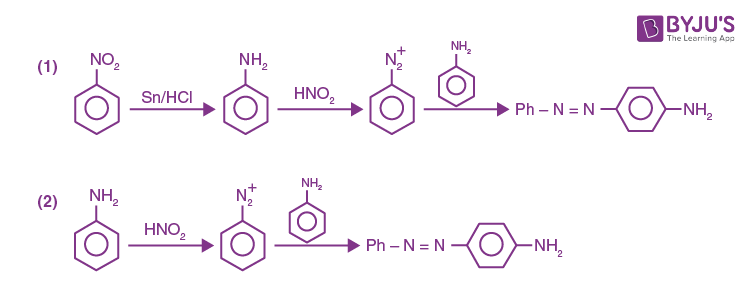Question 17. The hybridization and magnetic nature of [Mn(CN)6]4– and [Fe(CN)6]3–, respectively are:

a. d2sp3 and paramagnetic
b. sp3d2 and paramagnetic
c. d2sp3 and diamagnetic
d. sp3d2 and diamagnetic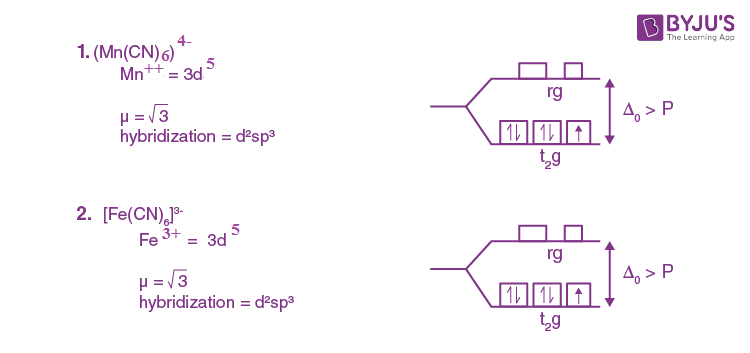Question 18. Identify A and B in the chemical reaction.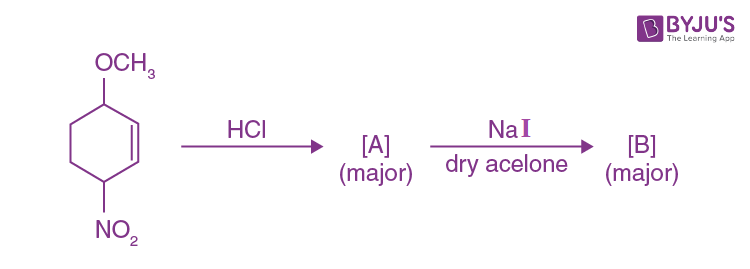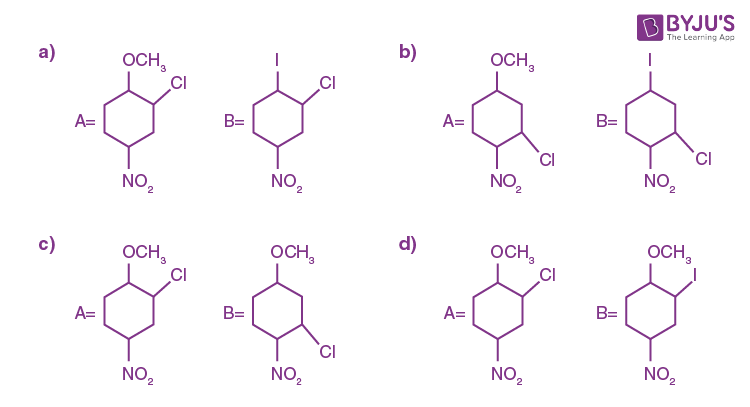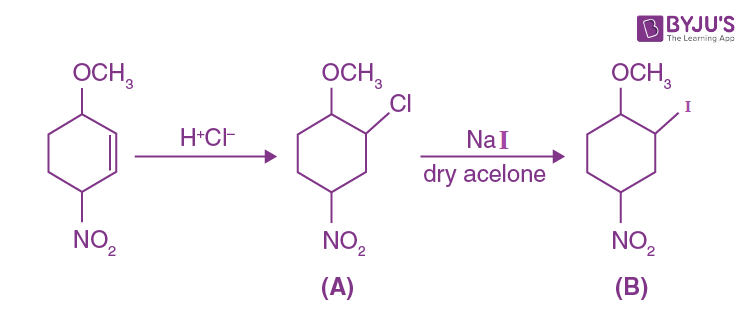Question 19. Compound(s) which will liberate carbon dioxide with sodium bicarbonate solution is/are: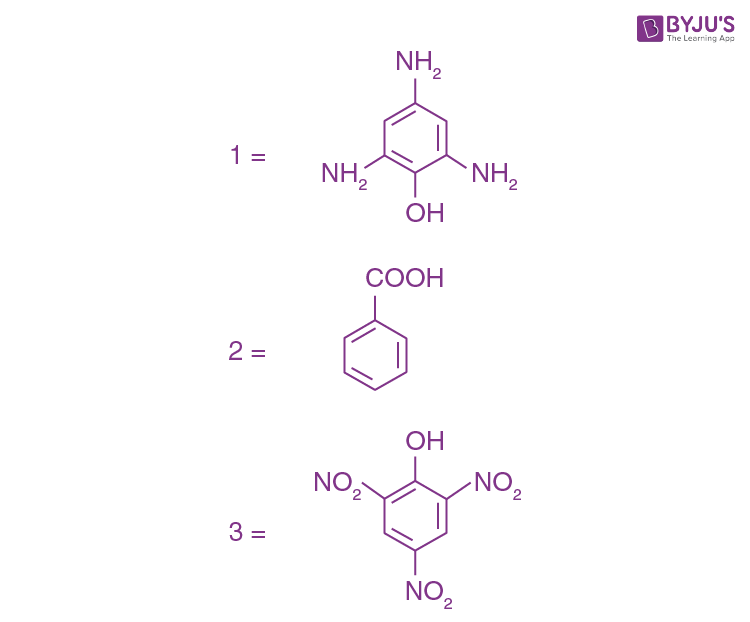a. 2 and 3 only
b. 1 only
c. 2 only
d. 3 only

Compounds that are more acidic than H2CO3, gives CO2 gas in reaction with NaHCO3. Compound B i.e. Benzoic acid and compound C i.e. picric acid both are more acidic than H2CO3.

Question 20. The solubility of AgCN in a buffer solution of pH = 3 is x. The value of x is: [Assume: No cyano complex is formed; Ksp(AgCN) = 2.2 × 10–16 and Ka (HCN) = 6.2 × 10–10]

a. 0.625 × 10-6
b. 1.6 × 10-6
c. 2.2 × 10-16
d. 1.9 × 10-5

Let solubility is x

AgCN ⇌ Ag+ + CNKsp = 2.2 × 10–16

x x

H+ + CN ⇌ HCN

$$\begin{array}{l}K = \frac{1}{K_{a}} = \frac{1}{6.2\times 10^{-10}}\end{array}$$

Ksp × 1/ka = [Ag+] [CN] × [HCN] / [H+][CN]

2.2 × 10-16 × 1 / 6.2 × 10-10 = [S][S] / 10-3

S2 = 2.2 / 6.2 × 10-9

S2 = 3.55 × 10–10

S = √3.55 × 10–10

S = 1.88 × 10–5 ⇒ 1.9 × 10–5

SECTION B

Question 1. The reaction of cyanamide, NH2CN(s) with oxygen was run in a bomb calorimeter and ΔU was found to be –742.24 kJ mol–1. The magnitude of ΔH298 for the reaction NH2CN (s) + 3/2 O2 (g) → N2 (g) + O2 (g) + H_2O(l) is______kJ. (Rounded off to the nearest integer). [Assume ideal gases and R = 8.314 J mol–1 K–1]

NH2CN(s) + 3/2 O2(g) → N2(g) + CO2(g) + H2O(λ)

Δng = (1 + 1) – 3/2 = ½

ΔH = ΔU + Δng RT

= -742.24 + ½ × 8.314 × 298 / 1000

= -742.24 + 1.24

= 741 kJ/mol

Question 2. In basic medium CrO42– oxidizes S2O32– to form SO24 and itself changes into Cr(OH)4. The volume of 0.154 M CrO42– required to react with 40 mL of 0.25 M S2O32– is ______ mL. (Rounded-off to the nearest integer)

17H2O + 8CrO4 + 3S2O3 → 6SO4 + 8Cr(OH)4 + 2OH

Applying mole-mole analysis

0.154 × v / 8 = 40 × 0.25 / 3

V = 173 mL

Question 3. For the reaction, aA + bB → cC + dD, the plot of log k vs 1/T is given below: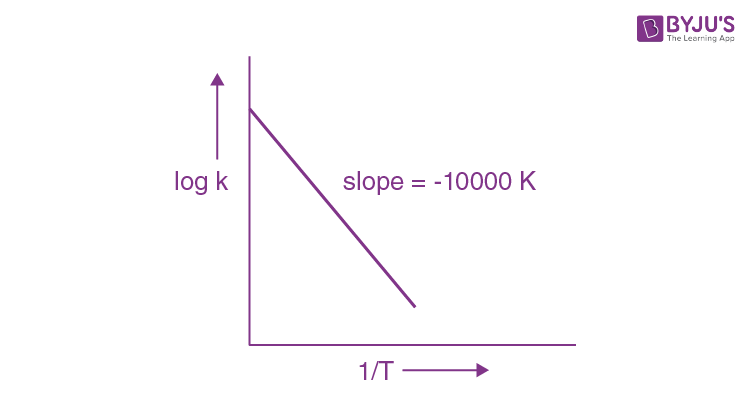The temperature at which the rate constant of the reaction is 10–4s–1 is ________ K. [Rounded off to the nearest integer)

[Given: The rate constant of the reaction is 10–5 s–1 at 500 K]

$$\begin{array}{l}log_{10}K = log_{10}A – \frac{E_{a}}{2.303RT}\end{array}$$
$$\begin{array}{l}Slope = \frac{E_{a}}{2.303R} = -10000\end{array}$$
$$\begin{array}{l}log_{10\frac{K_{1}}{K_{2}}} = \frac{E_{a}}{2.303R} \times \left ( \frac{1}{T_{1}} – \frac{1}{T_{2}} \right )\end{array}$$
$$\begin{array}{l}log_{10\frac{10^{-4}}{10^{-5}}} = 1000\times \left ( \frac{1}{500} – \frac{1}{T} \right )\end{array}$$
$$\begin{array}{l}1 = 1000\times \left ( \frac{1}{500} – \frac{1}{T} \right )\end{array}$$
$$\begin{array}{l}\frac{1}{10000} = \frac{1}{500} = \frac{1}{T}\end{array}$$
$$\begin{array}{l}\frac{1}{T} = \frac{1}{500} = \frac{1}{10000}\end{array}$$
$$\begin{array}{l}\frac{1}{T} = \frac{20-1}{10000} = \frac{19}{10000}\end{array}$$

T = 10000 / 19

T= 526 K

Question 4. 0.4g mixture of NaOH, Na2CO3 and some inert impurities was first titrated with N / 10HCl using phenolphthalein as an indicator, 17.5 mL of HCl was required at the end point. After this methyl orange was added and titrated. 1.5 mL of the same HCl was required for the next end point. The weight percentage of Na2CO3 in the mixture is ______. (Rounded-off to the nearest integer)

1st end point reaction

NaOH + HCl → NaCl + H2O

nf = 1

NaCO3 + HCl → NaHCO3

nf = 1

Eq of HCl used = nNaOH × 1 + nNa2CO3 × 1

17.5 × 1/10 × 10-3 = nNaOH + nNa2CO3

2nd end point

NaHCO3 + HCl → H2CO3

1.5 × 1/10 × 10-3 = nNaHCO3 × 1 = nNaHCO3

0.15 mmol = nNa2CO3

0.15 = nNa2CO3

$$\begin{array}{l}W_{Na_{2}CO_{3}} = \frac{0.15\times 106\times10^{-3} }{0.5} \times100 \times 10\end{array}$$

= 3 × 106 × 10–2

= 3 × 1.06 = 3.18%

Question 5. The ionization enthalpy of Na+ formation from Na(g) is 495.8 kJ mol–1, while the electron gain enthalpy of Br is –325.0 kJ mol–1. Given the lattice enthalpy of NaBr is –728.4 kJ mol–1. The energy for the formation of NaBr ionic solid is (–)_____ × 10–1 kJ mol–1.

Na(s) → Na+(g) ΔH = 495.8

½ Br2(λ) + e → Br(g) ΔH = 325

Na+(g) + Br(g) → NaBr(s) ΔH = –728.4

Na(s) + ½ Βr2(λ) → NaBr(s). ΔH =?

ΔH = 495.8 – 325 – 728.4

–557.6 kJ = –5576 × 10–1 kJ

Question 6. Consider the following chemical reaction.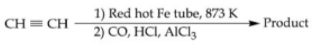The number of sp2 hybridized carbon atom(s) present in the product is _______.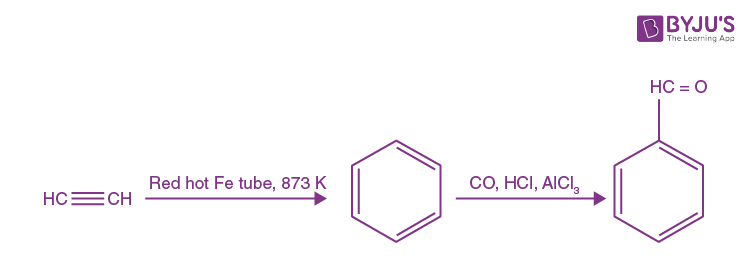All carbon atoms in benzaldehyde are sp2 hybridized.

Question 7. A car tire is filled with nitrogen gas at 35 psi at 27°C. It will burst if pressure exceeds 40 psi. The temperature in °C at which the car tyre will burst is ______. (Rounded-off to the nearest integer)

P1 / T1 = P2 / T2

35 / 300 = 40 / T2

T2 = 40 × 300 / 35

= 342.86 K

= 69.85°C ≃ 70°C

Question 8. Among the following, the number of halide(s) which is/are inert to hydrolysis is ______.

a. BF3
b. SiCl4
c. PCl5
d. SF6

Due to crowding, SF6 is not hydrolyzed.

Question 9. 1 molal aqueous solution of an electrolyte A2B3 is 60% ionised. The boiling point of the solution at 1 atm is _____ K. (Rounded-off to the nearest integer). [Given Kb for (H2O) = 0.52 K kg mol–1]

A2B3 → 2A+3 + 3B–2

No. of ions = 2 + 3 = 5

wi = 1 + (n – 1) ∝

= 1 + (5 – 1) × 0.6

= 1 + 4 × 0.6 = 1 + 2.4 = 3.4

ΔTb = Kb × m × i

= 0.52 × 1 × 3.4 = 1.768°C

ΔTb = (Tb)solution – [(TbH2O)]solution

1.768 = (Tb)solution – 100

(Tb)solution = 101.768 °C

= 375 K

Question 10. Using the provided information in the following paper chromatogram: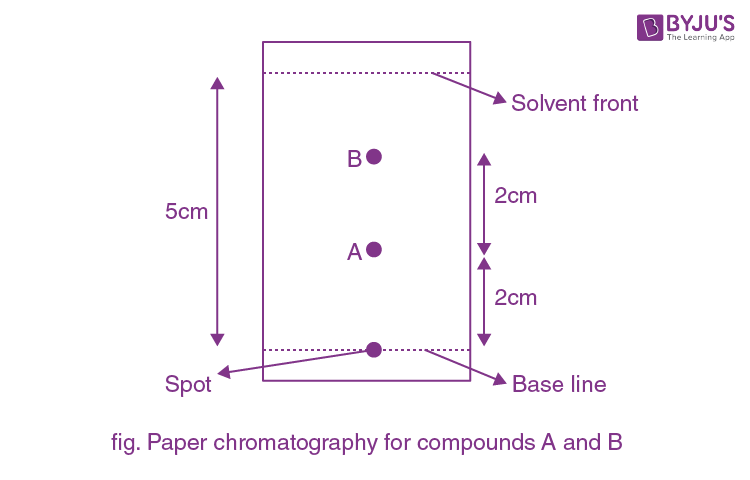The calculated Rf value of A ______ × 10–1.

$$\begin{array}{l}R_{r} = \frac{Distance\; travelled\; by\; compound}{Distance\; travelled\; by\; solvent }\end{array}$$

On chromatogram distance travelled by compound is → 2 cm

Distance travelled by solvent = 5 cm

So Rf = 2 / 5 = 4 × 10–1 = 0.4